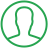# Import Class From Another File Python

Last Updated : Oct 16, 2023In this article we will show you the solution of import class from another file python, the equivalent of #include header_file in C/C++ is imported in Python.

Python modules can access code from other modules by using the import command to include the file or function.

The most popular method of using the import mechanism is by using an import statement, although it is not the only method.

The import keyword and the module's name make up the import statement. We will now discuss the idea of how to import a class from another file in python with an example.

## Step By Step Guide On Import Class From Another File Python :-

```import module
MyFile.py
class Square:
def __init__(self, val):
self.val = val
def getVal(self):
return self.val * self.val
return a + b
def sub(self, a, b):
return a - b
main.py
import MyFile
object1 = MyFile.Square(5)
print(f"From class Square: {object1.getVal()}")
print(f"From class Add_Sub: Subtraction {object2.sub(2, 3)}")```
1. The custom module is first imported by the code.
2. Then, two classes are defined by the code in a file named MyFile.py.
3. The first class is called Square, and its __init__ method accepts a value and initializes the instance variable val with it. There is a getVal method as well, which returns the square of the value.
4. Add_Sub is the second class, and it has the add and sub methods. When given two arguments, a and b, the add function delivers their total, whereas when given the same two arguments, a and b, the sub method returns their difference.
5. The MyFile module is imported by the code in the main.py file.
6. Then, an object object1 of the Square type is created with a starting value of 5. The square of the object's value is then printed using the getVal function of the Square class.
7. The process then produces an object2 of the Add_Sub class. It does addition using the add method and prints the outcome. Additionally, it subtracts using the sub technique and outputs the outcome.
8. The code comes to an end.

## Conclusion :-

As a result, we have successfully learned how to import a class from another file in python with an example.

Modular programming, a crucial component of effective programming, is implemented by importing classes and code from another file.

In this tutorial, we learned how to import classes both from and into the same directory.

No matter the language, importing classes and modules is the fundamental building block of programming.

I hope this article on import class from another file python helps you and the steps and method mentioned above are easy to follow and implement.

##About Dikshita

Passionate Electronics and Communication Engineering student with expertise in web development (HTML, CSS, JS,PHP, Bootstrap, React.js) and content writing. Eager problem solver and tech enthusiast, adept at creating engaging web experiences.